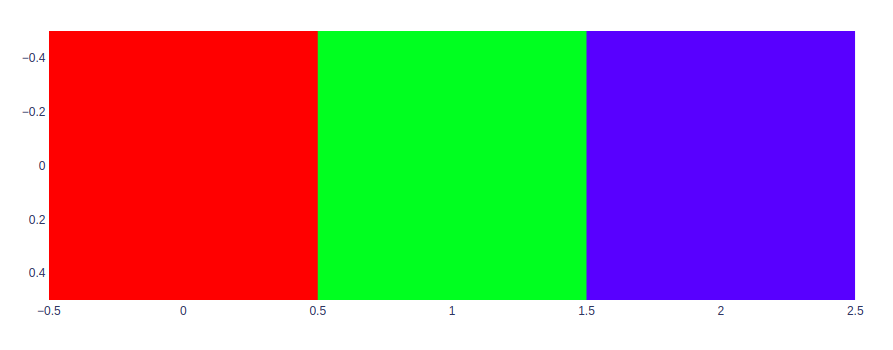Open in App
Not now

# How to display image using Plotly?

• Last Updated : 01 Oct, 2020

A Plotly is a Python library that is used to design graphs, especially interactive graphs. It can plot various graphs and charts like histogram, barplot, boxplot, spreadplot, and many more. It is mainly used in data analysis as well as financial analysis. plotly is an interactive visualization library.

## Displaying Image

The Imshow method is the fastest method to show the 2d data. This method is used to generate an image from the numerical data. The numerical data can be in the form of NumPy array.

Syntax: imshow(labels={}, x=None, y=None, color_continuous_scale=None, color_continuous_midpoint=None, range_color=None,width=None, height=None)

### Displaying RBG data as image

This function can also show RGB data as an image. The data is provided in the format of NumPy array.

Example:

## Python3

 `import` `plotly.express as px``import` `numpy as np`` ` ` ` `# RGB Data as numpy array``img_rgb ``=` `np.array([[[``255``, ``0``, ``0``], [``0``, ``255``, ``0``], [``0``, ``0``, ``255``]],``                    ``], dtype``=``np.uint8)`` ` `fig ``=` `px.imshow(img_rgb)``fig.show()`

Output:### Displaying image from image files

A third-party library can be used like PIL, scikit-image, or OpenCV to read the image as an array.

Example:

Image used:## Python3

 `import` `plotly.express as px``import` `cv2 ``   ` `# You can give path to the ``# image as first argument ``img ``=` `cv2.imread(``'GFG.png'``)`` ` `fig ``=` `px.imshow(img)``fig.show()`

Output:My Personal Notes arrow_drop_up